1、来自人民大会堂的一封邀请函

手机响起了熟悉的微信提示音，是一条活动邀请函，对于几乎每个星期都要参加各种活动的汽车媒体来说，这早已是司空见惯的事情，感觉就像老妈又叫我出去打酱油一样。

可是当我打开邀请函，打酱油的心情瞬间变成了买黄金的心情，即紧张又激动，因为这次活动地点不是在那些酒店的会议室，而是在北京的人民大会堂，刚刚举办完十九大的那个人民大会堂。这是我20多年来第一次不是拿着门票进入人民大会堂，也是第一次坐在人民大会堂参加会议，经常能在电视上看到的开会画面立马浮现在我脑海里。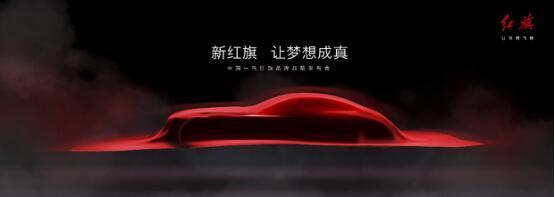（这是红旗战略发布会邀请函下的一张图，这是否意味着红旗将会发布一款超级跑车呢？1月8日会揭晓了。）

每年关乎中国人民未来命运的会议都会在这个人民大会堂举办，例如每年一次的全国人民代表大会、中国人民政治协商会议以及五年一届的中国共产党全国代表大会，它们描绘的是中华人民未来几年甚至十几年的宏伟蓝图。

而这次红旗在这里举办它们的战略发布会会如同那些大会一样是中国人的梦吗？虽然这场发布会要在1月8日才举行，可是我可以想象这绝对不是一场汽车品牌战略发布会那么简单，而是中国豪华汽车品牌崛起的起点，描绘的将会是中国豪华汽车品牌未来发展的宏伟蓝图。

我这个想象并不是空穴来风，从红旗邀请的出席嘉宾就可以看出一些端倪。一般的活动嘉宾除了厂家的领导外，就是各路的明星，无非就是唱歌跳舞，助助兴而已。

这一次红旗邀请的明星大咖几乎全是中国的骄傲，例如16年带领中国女排获得里约奥运会冠军的郎平；18岁就获得第14届肖邦国际钢琴比赛冠军的李云迪；凭借《秋雨》获得了雅尔塔国际电影节金奖的靳东；还有中国舞协第十届副主席黄豆豆。这些风云人物曾经帮中国获得各种荣耀，就像红旗作为国宾车承担起中国礼器的大任一样。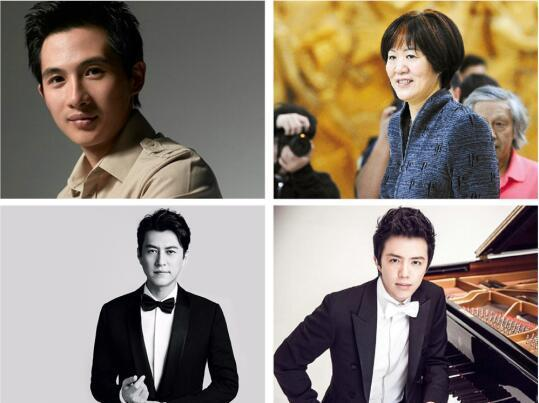2、这是一份历史的担当，同时也是中国汽车品牌“一哥”的气魄

前不久上映了一部电影，《血战湘江》，被剥夺了中央军事权力的毛泽东对着来自共产国际的李德和犯了严重左倾错误的博古大喊着：“我们红军子弟有能力把这些重新造出来！”这时候的红军子弟正经历着生与死的考验，拼了命地在护送背负笨重铁器的第二分队度过湘江。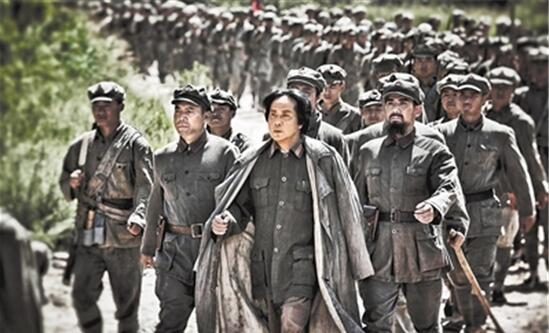毛泽东的这一句呐喊是中华民族站起来的底气，就凭这一口气，中国才有今时今日的成就。而在新中国成立之年，我们又听到了同样有底气的话，毛泽东参观完斯大林汽车厂之后说了一句：“我们也要有这样的工厂。”中国人的汽车梦就此开始！

1953年6月下旬，毛主席大手一挥，在一张八开的宣纸上写下“第一汽车制造厂奠基纪念”，中国的汽车梦就这样开始了，而我们常说的一汽就是第一汽车的简称，也就是“共和国汽车长子”，而长子自然也就承担起中国汽车白手起家的重任。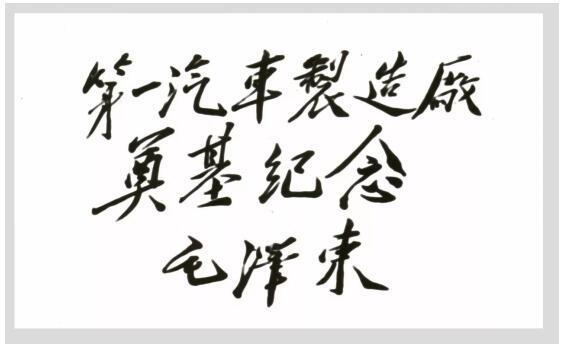当时研制国产轿车的条件堪称“四无”，即无资料、无经验、无工装、无设备，有的只是各位工匠的不屈精神以及任劳任怨的态度。整个车身的制造和各种钣金件的加工，几乎完全靠手工工艺敲打成型。当时，不少人吃住在生产现场，有的甚至把行李搬进车间，为的就是尽快实现中国人的汽车梦。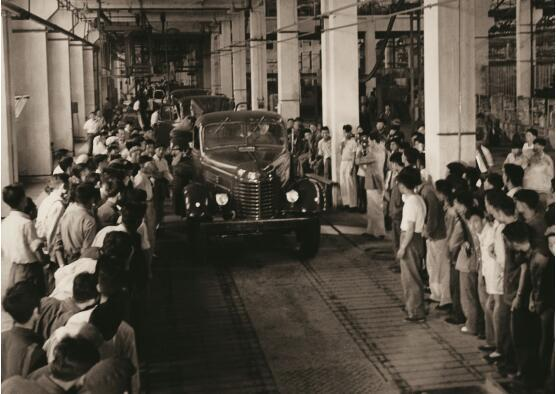就这样敲敲打打，中国的汽车工业从一无所有到现在占据了全球汽车销量的25%，而中国汽车品牌也占据了中国乘用车将近一半的销量，就在这么一个辉煌成绩的背后，中国汽车品牌依旧抛不开低价低质量的形象，永远靠性价比和合资品牌一决高下。

中国汽车不缺品牌，缺的是有质量的豪华品牌，这几年陆续有一些品牌开始品牌向上的路线。可是中国人对中国豪华品牌的态度就像是当年博古犯的左倾错误一样，总认为国外的月亮特别圆。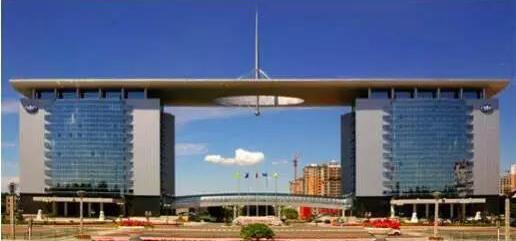（一汽曾被称为汽车业的共和国长子）

时至今日，中国汽车品牌依旧缺乏一个领军人物，而这个人物还是离不开中国汽车的长子，就像去年的8月2日，一汽董事长，徐留平，在履新当日的发言中的表态一样：“从国家、社会层面讲，一汽必须承载起“汽车强国梦”的重大责任。”如同60年前，一汽承当起全国人民的汽车梦一样。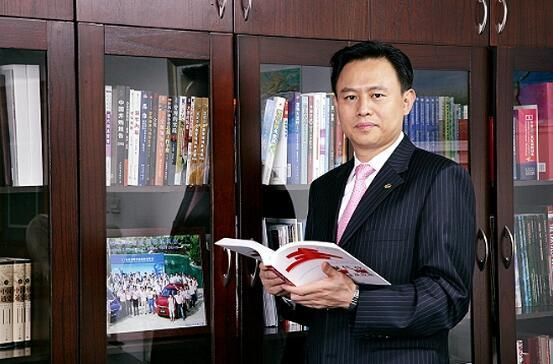所以，为什么1月8日的红旗战略发布会那么重要？因为60年前，红旗承担起了国事活动中的重要“礼器”，彰显着国之尊严和荣耀，重拾了礼仪之邦的威仪。而如今，在中国人渴望一个高品质的豪华品牌的时候，红旗汽车将会再次承载中国人的汽车梦，在1月8日晚上描绘中国豪华汽车品牌的宏伟蓝图，这也是一汽董事长徐留平直接管理红旗品牌的第一张发展蓝图。

目前红旗只发布了两个汽车平台的架构，一个是L系列的豪华车，另一个就是H系列的公商务车。此前红旗H7已经隆重上市，售价区间是24.98-47.98万，占据了中国市场的制高点，而且还没上市就已经获得了100台的大宗订单，不可谓不成功。

而元首级轿车——红旗L5也准备开始官网定购，售价600万元起，将会开启中国汽车的新高度。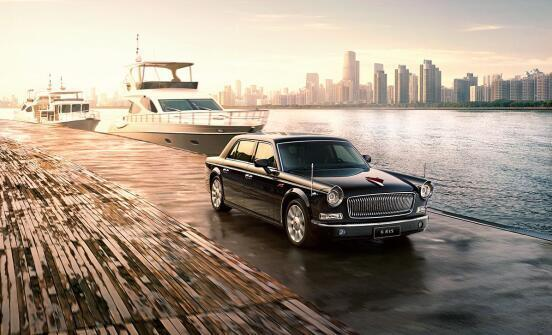虽然这次战略发布会还没有太多的信息透露出来，但是在去年12月份，红旗就表示未来会有搭载更多动力总成的红旗H7出来，例如红旗H7的混动版本，FCV以及纯电动车型，毋庸置疑，未来电动化和智能化将会是红旗的主路线。

另外，这次战略发布会除了会公布全新的设计语言之外，智能网联和未来的产品架构都是这次发布会的重点。遥想2012年的那一场红旗发布会，我们不仅看到了W12发动机、红旗L5和H7等等产品的出现，还看到了一个前所未有新高度的中国汽车品牌。

不管这次发布会的内容会是什么，红旗都会在“举全集团之力，多维度支持和打造红旗品牌”的方针政策下，我们都渴望看到红旗会带给我们什么惊喜。

正如徐留平在2017年最后一天发布的《2018年新年贺词》所说的一样：“2018年，是一汽落实‘四步走’战略、加快推进企业转型升级的基点之年。胸怀梦想，击鼓催征，扛红旗、抓自主、挺创新、强合作，我们将义无反顾，全力以赴。”（文/Dearauto）

##### 相关资讯

|分享

•已阅！握爪
•我手滑为你点赞
•128个赞！
•不明觉厉
•阅后既醉
•有钱！任性
•照片太美，我不敢看
•干货！杠杠的
•高大上
•膜拜中！
•请接受我的膝盖
•猫哥/猫妹么么哒
•神吐槽
•我只笑笑不说话
•我想静静
•窒息
##### 资讯相关车系##### 热度排行• 阿斯顿·马丁
• 奥迪
• 阿尔法罗密欧
• ALPINA
• 爱驰
• AC Schnitzer

• 巴博斯
• 宝骏
• 宝马
• 保时捷
• 北汽制造
• 奔驰
• 奔腾
• 本田
• 比亚迪
• 标致
• 别克
• 宾利
• 布加迪
• 北汽威旺
• 北京
• 北汽绅宝
• 北汽幻速
• 北汽新能源
• 宝沃
• 比速汽车
• 北汽道达
• 北京汽车
• 比德文汽车
• 铂驰
• 北汽昌河

• 昌河
• 长安
• 长城
• 长安商用
• 成功汽车
• 长江EV
• 长安轻车型
• 长安欧尚
• 长安凯程
• 长安跨越
• 车驰汽车

• 大众
• 道奇
• 东风
• 东风风神
• 东风小康
• 东南
• DS
• 东风风行
• 东风风度
• 东风风光
• 电咖
• 大乘汽车
• 大运
• 东风富康

• 法拉利
• 菲亚特
• 丰田
• 福迪
• 福特
• 福田汽车
• 福汽启腾
• 风诺
• 福田

• GMC
• 广汽传祺
• 广汽吉奥
• 观致
• 国金汽车
• 广汽新能源
• 广汽集团
• 国机智骏

• 哈飞
• 海格
• 海马
• 华泰
• 黄海
• 恒天
• 红旗
• 哈弗
• 华颂
• 华凯
• 华泰新能源
• 汉腾汽车
• 华利
• 红星汽车
• HYCAN合创
• 汉龙汽车

• Jeep
• 江淮
• 江铃
• 捷豹
• 金杯
• 九龙
• 吉利汽车
• 金旅
• 金龙
• 江铃集团轻汽
• 江铃集团新能源
• 君马汽车
• 捷途
• 钧天
• 捷达
• 几何汽车

• 开瑞
• 凯迪拉克
• 科尼赛克
• 克莱斯勒
• KTM
• 卡威
• 凯翼
• 康迪
• 康迪电动汽车集团
• 开沃汽车
• 卡升

• 猎豹汽车
• 兰博基尼
• 劳斯莱斯
• 雷克萨斯
• 雷诺
• 理念
• 力帆
• 莲花汽车
• 林肯
• 铃木
• 陆风
• 路虎
• 路特斯
• 领志
• 领克
• 零跑汽车
• Lorinser
• LOCAL MOTORS
• LITE
• 领途汽车
• 理想汽车
• 罗夫哈特
• 力帆汽车

• MG
• MINI
• 马自达
• 玛莎拉蒂
• 迈凯伦
• 摩根
• 名爵
• 迈莎锐
• 迈迈

• 纳智捷
• 哪吒汽车

• 讴歌
• 欧宝
• 欧朗
• 欧拉
• 欧尚汽车

• 帕加尼
• Polestar极星

• 奇瑞
• 启辰
• 起亚
• 前途
• 庆铃汽车
• 骐铃汽车
• 全球鹰
• 乔治·巴顿

• 日产
• 荣威
• 瑞麒汽车
• 如虎
• 瑞驰
• 瑞麒
• 瑞驰新能源
• 容大智造

• 上汽大通
• smart
• 三菱
• 双环
• 双龙
• 斯巴鲁
• 斯柯达
• 萨博
• 思铭
• 赛麟
• SWM斯威汽车
• 上汽MAXUS
• SRM鑫源
• 思皓
• SHELBY
• 上喆

• TESLA
• 泰卡特
• 腾势
• 特斯拉
• 天际汽车

• 威麟
• 威兹曼
• 沃尔沃
• 五菱汽车
• 五十铃
• 潍柴英致
• WEY
• 蔚来
• 威马汽车
• 潍柴汽车

• 现代
• 雪佛兰
• 雪铁龙
• 西雅特
• 新特汽车
• 小鹏汽车
• 新宝骏

• 野马汽车
• 一汽
• 依维柯
• 英菲尼迪
• 永源
• 驭胜
• 云度
• 宇通客车
• 云雀汽车
• 远程汽车
• 一汽凌河

• 中华
• 中兴
• 众泰
• 知豆
• 之诺
• 正道汽车
• A
• B
• C
• D
• E
• F
• G
• H
• I
• J
• K
• L
• M
• N
• O
• P
• Q
• R
• S
• T
• U
• V
• W
• X
• Y
• Z

• 阿斯顿·马丁
• 奥迪
• 阿尔法罗密欧
• ALPINA
• 爱驰
• AC Schnitzer

• 巴博斯
• 宝骏
• 宝马
• 保时捷
• 北汽制造
• 奔驰
• 奔腾
• 本田
• 比亚迪
• 标致
• 别克
• 宾利
• 布加迪
• 北汽威旺
• 北京
• 北汽绅宝
• 北汽幻速
• 北汽新能源
• 宝沃
• 比速汽车
• 北汽道达
• 北京汽车
• 比德文汽车
• 铂驰
• 北汽昌河

• 昌河
• 长安
• 长城
• 长安商用
• 成功汽车
• 长江EV
• 长安轻车型
• 长安欧尚
• 长安凯程
• 长安跨越
• 车驰汽车

• 大众
• 道奇
• 东风
• 东风风神
• 东风小康
• 东南
• DS
• 东风风行
• 东风风度
• 东风风光
• 电咖
• 大乘汽车
• 大运
• 东风富康

• 法拉利
• 菲亚特
• 丰田
• 福迪
• 福特
• 福田汽车
• 福汽启腾
• 风诺
• 福田

• GMC
• 广汽传祺
• 广汽吉奥
• 观致
• 国金汽车
• 广汽新能源
• 广汽集团
• 国机智骏

• 哈飞
• 海格
• 海马
• 华泰
• 黄海
• 恒天
• 红旗
• 哈弗
• 华颂
• 华凯
• 华泰新能源
• 汉腾汽车
• 华利
• 红星汽车
• HYCAN合创
• 汉龙汽车

• Jeep
• 江淮
• 江铃
• 捷豹
• 金杯
• 九龙
• 吉利汽车
• 金旅
• 金龙
• 江铃集团轻汽
• 江铃集团新能源
• 君马汽车
• 捷途
• 钧天
• 捷达
• 几何汽车

• 开瑞
• 凯迪拉克
• 科尼赛克
• 克莱斯勒
• KTM
• 卡威
• 凯翼
• 康迪
• 康迪电动汽车集团
• 开沃汽车
• 卡升

• 猎豹汽车
• 兰博基尼
• 劳斯莱斯
• 雷克萨斯
• 雷诺
• 理念
• 力帆
• 莲花汽车
• 林肯
• 铃木
• 陆风
• 路虎
• 路特斯
• 领志
• 领克
• 零跑汽车
• Lorinser
• LOCAL MOTORS
• LITE
• 领途汽车
• 理想汽车
• 罗夫哈特
• 力帆汽车

• MG
• MINI
• 马自达
• 玛莎拉蒂
• 迈凯伦
• 摩根
• 名爵
• 迈莎锐
• 迈迈

• 纳智捷
• 哪吒汽车

• 讴歌
• 欧宝
• 欧朗
• 欧拉
• 欧尚汽车

• 帕加尼
• Polestar极星

• 奇瑞
• 启辰
• 起亚
• 前途
• 庆铃汽车
• 骐铃汽车
• 全球鹰
• 乔治·巴顿

• 日产
• 荣威
• 瑞麒汽车
• 如虎
• 瑞驰
• 瑞麒
• 瑞驰新能源
• 容大智造

• 上汽大通
• smart
• 三菱
• 双环
• 双龙
• 斯巴鲁
• 斯柯达
• 萨博
• 思铭
• 赛麟
• SWM斯威汽车
• 上汽MAXUS
• SRM鑫源
• 思皓
• SHELBY
• 上喆

• TESLA
• 泰卡特
• 腾势
• 特斯拉
• 天际汽车

• 威麟
• 威兹曼
• 沃尔沃
• 五菱汽车
• 五十铃
• 潍柴英致
• WEY
• 蔚来
• 威马汽车
• 潍柴汽车

• 现代
• 雪佛兰
• 雪铁龙
• 西雅特
• 新特汽车
• 小鹏汽车
• 新宝骏

• 野马汽车
• 一汽
• 依维柯
• 英菲尼迪
• 永源
• 驭胜
• 云度
• 宇通客车
• 云雀汽车
• 远程汽车
• 一汽凌河

• 中华
• 中兴
• 众泰
• 知豆
• 之诺
• 正道汽车
• A
• B
• C
• D
• E
• F
• G
• H
• I
• J
• K
• L
• M
• N
• O
• P
• Q
• R
• S
• T
• U
• V
• W
• X
• Y
• Z

• 阿斯顿·马丁
• 奥迪
• 阿尔法罗密欧
• ALPINA
• 爱驰
• AC Schnitzer

• 巴博斯
• 宝骏
• 宝马
• 保时捷
• 北汽制造
• 奔驰
• 奔腾
• 本田
• 比亚迪
• 标致
• 别克
• 宾利
• 布加迪
• 北汽威旺
• 北京
• 北汽绅宝
• 北汽幻速
• 北汽新能源
• 宝沃
• 比速汽车
• 北汽道达
• 北京汽车
• 比德文汽车
• 铂驰
• 北汽昌河

• 昌河
• 长安
• 长城
• 长安商用
• 成功汽车
• 长江EV
• 长安轻车型
• 长安欧尚
• 长安凯程
• 长安跨越
• 车驰汽车

• 大众
• 道奇
• 东风
• 东风风神
• 东风小康
• 东南
• DS
• 东风风行
• 东风风度
• 东风风光
• 电咖
• 大乘汽车
• 大运
• 东风富康

• 法拉利
• 菲亚特
• 丰田
• 福迪
• 福特
• 福田汽车
• 福汽启腾
• 风诺
• 福田

• GMC
• 广汽传祺
• 广汽吉奥
• 观致
• 国金汽车
• 广汽新能源
• 广汽集团
• 国机智骏

• 哈飞
• 海格
• 海马
• 华泰
• 黄海
• 恒天
• 红旗
• 哈弗
• 华颂
• 华凯
• 华泰新能源
• 汉腾汽车
• 华利
• 红星汽车
• HYCAN合创
• 汉龙汽车

• Jeep
• 江淮
• 江铃
• 捷豹
• 金杯
• 九龙
• 吉利汽车
• 金旅
• 金龙
• 江铃集团轻汽
• 江铃集团新能源
• 君马汽车
• 捷途
• 钧天
• 捷达
• 几何汽车

• 开瑞
• 凯迪拉克
• 科尼赛克
• 克莱斯勒
• KTM
• 卡威
• 凯翼
• 康迪
• 康迪电动汽车集团
• 开沃汽车
• 卡升

• 猎豹汽车
• 兰博基尼
• 劳斯莱斯
• 雷克萨斯
• 雷诺
• 理念
• 力帆
• 莲花汽车
• 林肯
• 铃木
• 陆风
• 路虎
• 路特斯
• 领志
• 领克
• 零跑汽车
• Lorinser
• LOCAL MOTORS
• LITE
• 领途汽车
• 理想汽车
• 罗夫哈特
• 力帆汽车

• MG
• MINI
• 马自达
• 玛莎拉蒂
• 迈凯伦
• 摩根
• 名爵
• 迈莎锐
• 迈迈

• 纳智捷
• 哪吒汽车

• 讴歌
• 欧宝
• 欧朗
• 欧拉
• 欧尚汽车

• 帕加尼
• Polestar极星

• 奇瑞
• 启辰
• 起亚
• 前途
• 庆铃汽车
• 骐铃汽车
• 全球鹰
• 乔治·巴顿

• 日产
• 荣威
• 瑞麒汽车
• 如虎
• 瑞驰
• 瑞麒
• 瑞驰新能源
• 容大智造

• 上汽大通
• smart
• 三菱
• 双环
• 双龙
• 斯巴鲁
• 斯柯达
• 萨博
• 思铭
• 赛麟
• SWM斯威汽车
• 上汽MAXUS
• SRM鑫源
• 思皓
• SHELBY
• 上喆

• TESLA
• 泰卡特
• 腾势
• 特斯拉
• 天际汽车

• 威麟
• 威兹曼
• 沃尔沃
• 五菱汽车
• 五十铃
• 潍柴英致
• WEY
• 蔚来
• 威马汽车
• 潍柴汽车

• 现代
• 雪佛兰
• 雪铁龙
• 西雅特
• 新特汽车
• 小鹏汽车
• 新宝骏

• 野马汽车
• 一汽
• 依维柯
• 英菲尼迪
• 永源
• 驭胜
• 云度
• 宇通客车
• 云雀汽车
• 远程汽车
• 一汽凌河

• 中华
• 中兴
• 众泰
• 知豆
• 之诺
• 正道汽车
• A
• B
• C
• D
• E
• F
• G
• H
• I
• J
• K
• L
• M
• N
• O
• P
• Q
• R
• S
• T
• U
• V
• W
• X
• Y
• Z